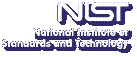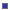Up# Small Circuits for Boolean Functions

Rene C. Peralta
NIST, Computer Security Division (893)

Tuesday, August 25, 2009 15:00-16:00,
Building 101, Employee Lounge
Gaithersburg
Tuesday, August 25, 2009 13:00-14:00,
Room 5000
Boulder

Abstract: We consider the problem of building efficient circuits using arithmetic modulo 2. In this type of arithmetic, the only possible values are 0 and 1. Addition and multiplication are defined by x + x = 0 and x * x = x. If we identify the Boolean constants (TRUE,FALSE) with (1,0), then all Boolean logic can be encoded using arithmetic modulo 2: addition corresponds to logical XOR, multiplication to AND, and negation is given by NOT(x) = x + 1.

The problem of building efficient circuits is highly intractable for any meaningful measure of "efficiency". Thus, we can only hope to develop heuristics. We propose the following approach:find a circuit with as few multiplications as possible; thenoptimize the linear pieces of this circuit.

The multiplicative complexity of a function is the number of multiplications necessary and sufficient to compute it.

For example, the function:

f(x1,x2,x3) = x1 x2 + x1 x3 + x2 x3

can be computed with only one multiplication:

f(x1,x2,x3) = (x1 + x2)(x1 + x3) + x1.

(If you thought that was too easy then try computing

f(x1,x2,x3,x4) = x1 x2 x3 x4 + x1 x2 x3 + x1 x2 + x1 x3 + x1 x4 + x2 x3 + x2 x4 + x3 x4

using only 3 multiplications.)

I will discuss known bounds on the multiplicative complexity of functions. Some of these results are constructive, and thus can be used in the first step of our circuit optimization method.

The second part of the talk considers the problem of optimizing the linear parts of a circuit. In this problem, we are given a binary m-by-n matrix M, and the task is to build a circuit for computing Mx (where x is an n-bit input). It turns out most work in this area makes an implicit assumption that turns out to be false. When one sheds this assumption, it becomes clear that new heuristics are needed. We have coded one such heuristic. I will report on how it fares compared to traditional methods.

NIST, together with the University of Southern Denmark, are seeking patents on some of this work. Here are a couple of circuits mentioned in the patent application (red is multiplication, yellow is addition, gray is XNOR(x,y) = x + y + 1):

Speaker Bio: Rene Peralta's primary research interests are in mathematical cryptology and its applications. He received a B.A. in Economics from Hamilton College in 1978 and an M.A. in Mathematics from SUNY Binghamton in 1979. He received a Ph.D. in Computer Science from the University of California at Berkeley in 1985. He has held teaching and research positions at a number of academic institutions in the U.S, Chile, Japan, and Europe. In the 1990s he was Director of the Center for Cryptography, Computer, and Network Security at the University of Wisconsin, Milwaukee. In 2005, following a five-year teaching and research stay at Yale University, he joined the Computer Security Division of the Information Technology Laboratory at the National Institute of Standards and Technology.

Note: Visitors from outside NIST must contact Robin Bickel; (301) 975-3668; at least 24 hours in advance.